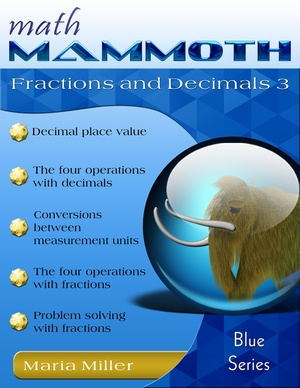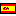^
You are here: HomeBlue Series → Fractions & Decimals 3

# Math Mammoth Fractions & Decimals 3107 pages

## Prices & ordering

Printed copy \$10.90 USDEste libro en español

Math Mammoth Fractions and Decimals 3 continues the study of fraction and decimal topics on the 6th grade level. This book assumes the student already has studied fractions and decimals in the past, for example using Math Mammoth Fractions 1, Math Mammoth Fractions 2 and Math Mammoth Decimals 2, or Math Mammoth Grade 5 Complete Curriculum.

The goal of the book is to go through all of the fraction and decimal arithmetic using up to six decimal digits and larger denominators in fractions than in 4th and 5th grade materials. For some students, this may mean a lot of review, so if the student already has a good grasp of these topics, consider assigning only one-fourth to one-half of the exercises initially. Monitor the student’s progress and assign more if needed. The skipped problems can be used for review later.

First we study all four operations of decimals, the metric system, and using decimals with measuring units. We start out by studying place value with decimals and comparing decimals up to six decimal digits. The next several lessons contain a lot of review, just using longer decimals than in fifth grade: adding and subtracting decimals, rounding decimals, multiplying and dividing decimals, fractions and decimals, and multiplying and dividing decimals by the powers of ten.

Since the chapter focuses on restudying the mechanics of decimal arithmetic, it is a good time to stress to your student(s) the need for accurate calculations and for checking one’s final answer. Notice how the lessons often ask students to estimate the answer before calculating the exact answer. Estimation can be used as a type of check for the final answer: if the final answer is far from the estimation, there is probably an error in the calculation. It can also be used to check if an answer calculated with a calculator is likely correct.

In the lessons about multiplication and division of decimals, students work both with mental math and with standard algorithms. The lessons that focus on mental math point out various patterns and shortcuts for students, helping them to see the structure and logic in math. I have also explained why the common rules (or shortcuts) for decimal multiplication and decimal division actually work, essentially providing a mathematical proof on a level that sixth graders can hopefully understand.

The last lessons deal with measuring units and the metric system, rounding out our study of decimals.

After decimals, the book covers all fraction arithmetic. We begin with a review of fraction arithmetic from fifth grade—specifically, addition, subtraction, simplification, and multiplication of fractions. Then the lessons focus on division of fractions.

The introductory lesson on the division of fractions presents the concept of reciprocal numbers and ties the reciprocity relationship to the idea that division is the appropriate operation to solve questions of the form, “How many times does this number fit into that number?” For example, we can write a division from the question, “How many times does 1/3 fit into 1?” The answer is, obviously, 3 times. So we can write the division 1 ÷ (1/3) = 3 and the multiplication 3 · (1/3) = 1. These two numbers, 3/1 and 1/3, are reciprocal numbers because their product is 1.

Students learn to solve questions like that through using visual models and writing division sentences that match them. Thinking of fitting the divisor into the dividend (measurement division) also gives us a tool to check whether the answer to a division problem is reasonable.

Naturally, the lessons also present the shortcut for fraction division—that each division can be changed into a multiplication by taking the reciprocal of the divisor, which is often called the “invert (flip)-and-multiply” rule. However, that “rule” is just a shortcut. It is necessary to memorize it, but memorizing a shortcut doesn’t help students make sense conceptually out of the division of fractions—they also need to study the concept of division and use visual models to better understand the process involved.

In two lessons that follow, students apply what they have learned to solve problems involving fractions or fractional parts. A lot of the problems in these lessons are review in the sense that they involve previously learned concepts and are similar to problems students have solved earlier, but many involve the division of fractions, thus incorporating the new concept presented in this book.

The PDF version of this book can be filled in on a computer, phone, or tablet, using the annotation tools found in many PDF apps. See more.

You might also be interested in:

## Better Yet - Bundle Deals!

*BONUS*: Buy any bundle listed above, and get the fully upgraded Soft-Pak software for FREE (includes 4 math and 2 language arts programs, complete with on screen and printable options).

## How and where to order

You can buy Math Mammoth books at:

• Here at MathMammoth.com website — simply use the "Add to cart" buttons you see on the product pages.
• Rainbow Resource carries printed copies for the Light Blue series books, plus several CDs (Light Blue and Blue series).
• Homeschool Buyers Club offers download versions of the Light Blue series, plus the Blue series bundle.
• Homeschool Planet sells the digital versions of the main curriculum and lesson plans to go with them.
• Lulu sells printed copies for most of the Math Mammoth materials (various series).

By purchasing any of the books, permission IS granted for the teacher (or parent) to reproduce this material to be used with his/her students in a teaching situation; not for commercial resale. However, you are not permitted to share the material with another teacher.

In other words, you are permitted to make copies for the students/children you are teaching, but not for other teachers' usage.

Math Mammoth books are PDF files. I recommend you use Adobe Reader to view them, including if you use a Mac. You can try other PDF viewers, but they may at times either omit or mess up some of the images.
WAIT!

Receive my monthly collection of math tips & resources directly in your inbox — and get a FREE Math Mammoth book!You can unsubscribe at any time.

### Math Mammoth Tour

Confused about the different options? Take a virtual email tour around Math Mammoth! You'll receive:

An initial email to download your GIFT of over 400 free worksheets and sample pages from my books. Six other "TOURSTOP" emails that explain the important things and commonly asked questions concerning Math Mammoth curriculum. (Find out the differences between all these different-colored series!)

This way, you'll have time to digest the information over one or two weeks, plus an opportunity to ask me personally about the curriculum.
A monthly collection of math teaching tips & Math Mammoth updates (unsubscribe any time)### "Mini" Math Teaching Course

This is a little "virtual" 2-week course, where you will receive emails on important topics on teaching math, including:

- How to help a student who is behind
- Troubles with word problems
- Teaching multiplication tables
- Why fractions are so difficult
- The value of mistakes
- Should you use timed tests
- And more!

A GIFT of over 400 free worksheets and sample pages from my books right in the very beginning.A monthly collection of math teaching tips & Math Mammoth updates (unsubscribe any time)
Enter your email to receive math teaching tips, resources, Math Mammoth news & sales, humor, and more! I tend to send out these tips about once monthly, near the beginning of the month, but occasionally you may hear from me twice per month (and sometimes less often).• A GIFT of over 400 free worksheets and sample pages from my books.